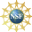# 8. Properties of Curves

## 2. Examples of Curve Computations

Here are summaries of the complete computations for the 3 curves we have looked at throughout this chapter: the circle, the helix and the twisted cubic, plus the computations for a new curve, the cubic spiral helix. Then in the exercises, you will be asked to compute everything for several other curves.

### Curve Exercises

Pick one of the following curves:

1. $$\vec{r}(t)=(e^{t}\cos t,e^{t}\sin t,e^{t})$$
2. $$\vec{r}(t)=(3t^2,4t^3,3t^4)$$
3. $$\vec{r}(t)=(e^{t},\sqrt{2}t,e^{-t})$$
4. $$\vec{r}(t)=\langle t^2,2t,\ln(t)\rangle$$
5. $$\vec{r}(t)=(\sinh(t),\cosh(t),t)$$
where $$\sinh(t)=\dfrac{e^t-e^{-t}}{2}$$ and $$\cosh(t)=\dfrac{e^t+e^{-t}}{2}$$.

Compute all of the following:

1. Velocity Vector: $$\vec{v}(t)=\dfrac{d\vec{r}}{dt}$$
2. Acceleration Vector: $$\vec{a}(t)=\dfrac{d\vec{v}}{dt}$$
3. Jerk Vector: $$\vec{j}(t)=\dfrac{d\vec{a}}{dt}$$
4. Speed: $$\dfrac{ds}{dt}=|\vec{v}|$$
5. Arclength from $$\vec{r}(0)$$ to $$\vec{r}(1)$$: $$\displaystyle L=\int_{\vec{r}(0)}^{\vec{r}(1)} ds=\int_0^1 |\vec{v}|\,dt$$
6. Unit Tangent Vector: $$\hat{T}=\dfrac{\vec{v}}{|\vec{v}|}$$
7. Velocity $$\times$$ Acceleration: $$\vec{v}\times\vec{a}\dfrac{}{}$$
8. its Length: $$|\vec{v}\times\vec{a}|\dfrac{}{}$$
9. Unit Binormal Vector: $$\hat{B}=\dfrac{\vec{v}\times\vec{a}}{|\vec{v}\times\vec{a}|}$$
10. Unit Normal Vector: $$\hat{N}=\hat{B}\times\hat{T}=\dfrac{\hat{T}'(t)}{\;|\hat{T}'(t)|\;}$$
11. Curvature: $$\kappa=\dfrac{|\vec{v}\times\vec{a}|}{|\vec{v}|^{3}} =\dfrac{\;|\hat{T}'(t)|\;}{|\vec{v}|}$$
12. Torsion: $$\tau=\dfrac{\vec{v}\times\vec{a}\cdot\vec{j}}{|\vec{v}\times\vec{a}|^2}$$
13. Tangential Acceleration: $$a_{T}=\vec{a}\cdot\hat{T}=\dfrac{d}{dt}|\vec{v}|$$
(Compute $$2$$ ways.)
14. Normal Acceleration: $$a_{N}=\vec{a}\cdot\hat{N}=\kappa|\vec{v}|^2=\dfrac{|\vec{v}|^2}{R}$$
(Compute $$2$$ ways.)

We have intentionally not given the solutions to these exercises so that they can be assigned as a take-home quiz.

Supported in part by NSF Grant #1123255